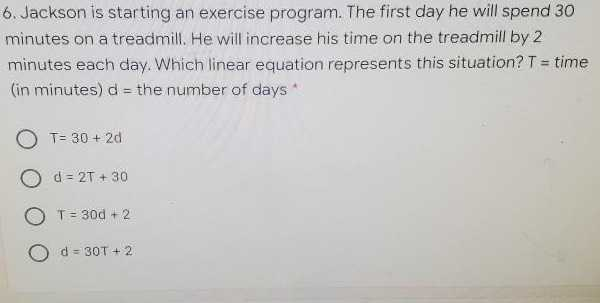### ¿Todavía tienes preguntas de matemáticas?

Pregunte a nuestros tutores expertos
Algebra
Pregunta6. Jackson is starting an exercise program. The first day he will spend $$30$$ minutes on a treadmill. He will increase his time on the treadmill by $$2$$ minutes each day. Which linear equation represents this situation? $$T =$$ time (in minutes) $$d =$$ the number of days

$$T = 30 + 2 d$$

$$d = 2 T + 30$$

$$T = 30 d + 2$$

$$d = 30 T + 2$$